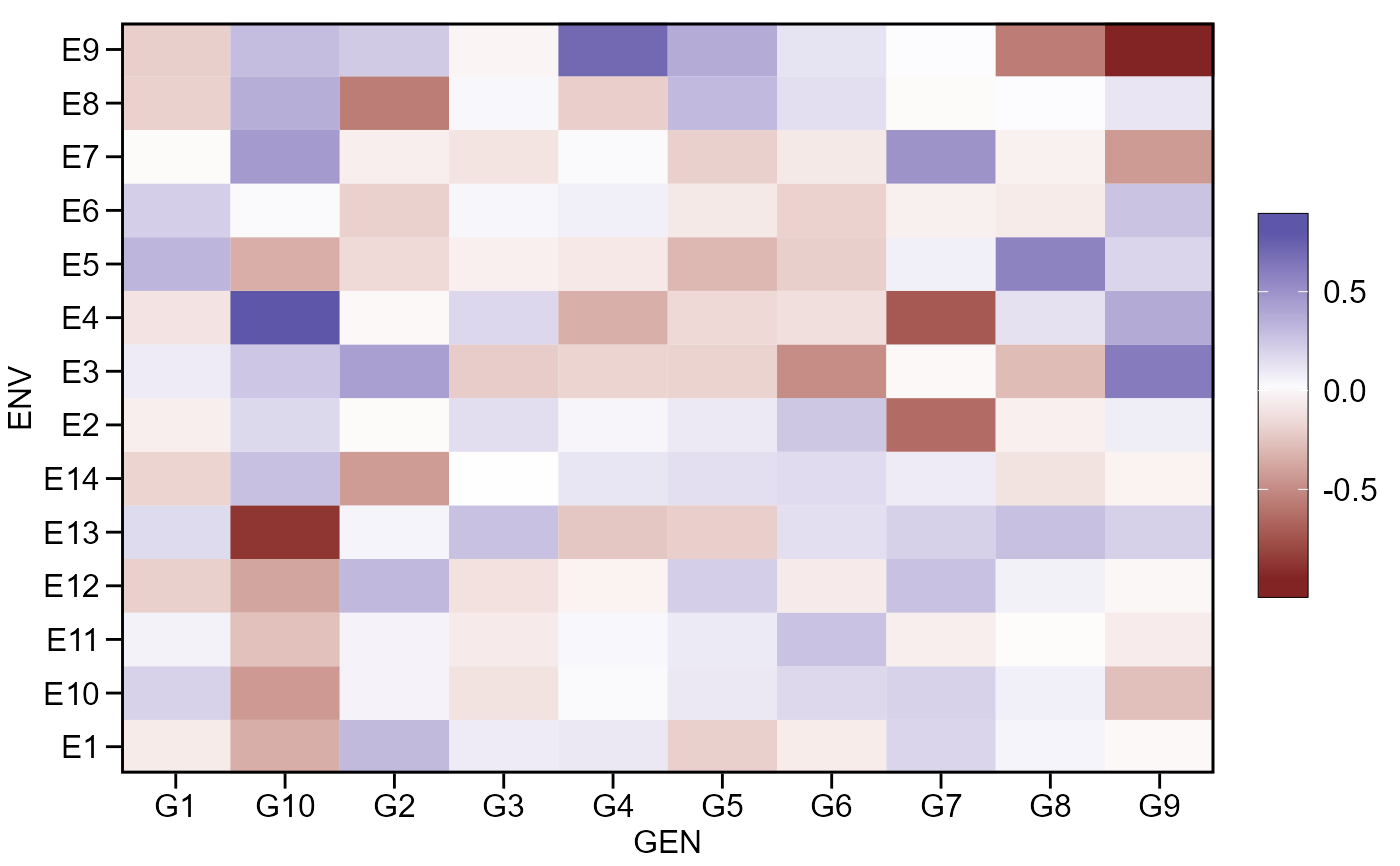This is a helper function that computes the genotype-environment effects, i.e., the residual effect of the additive model

## Usage

ge_effects(.data, env, gen, resp, type = "ge", verbose = TRUE)

## Arguments

.data

The dataset containing the columns related to Environments, Genotypes, replication/block and response variable(s).

env

The name of the column that contains the levels of the environments. The analysis of variance is computed for each level of this factor.

gen

The name of the column that contains the levels of the genotypes.

resp

The response variable(s). To analyze multiple variables in a single procedure a vector of variables may be used. For example resp = c(var1, var2, var3).

type

The type of effect to compute. Defaults to "ge", i.e., genotype-environment. To compute genotype plus genotype-environment effects use type = "gge".

verbose

Logical argument. If verbose = FALSE the code will run silently.

## Value

A list where each element is the result for one variable that contains a two-way table with genotypes in rows and environments in columns.

## Author

Tiago Olivoto tiagoolivoto@gmail.com

## Examples

# \donttest{
library(metan)
ge_eff <- ge_effects(data_ge, ENV, GEN, GY)
gge_eff <- ge_effects(data_ge, ENV, GEN, GY, type = "gge")
plot(ge_eff)# }